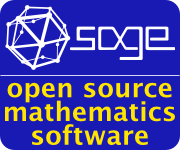# Sunil Chetty

 Associate Professor Mathematics Department College of Saint Benedict and Saint John's University Email:Office: MAIN 206 (CSB campus) Office Phone: 320-363-5960### My Info and News

I am an associate professor in the math department at the College of Saint Benedict/Saint John's University in central Minnesota.

From 2009-2011, I was a (post-doctoral) Riley scholar in the department of mathematics and computer science  at Colorado College (CC).

#### Teaching (please feel free to contact for details)

Current Courses
2021 Spring - Math 121: Math for Elementary Education 1
2021 Spring - Math 180: Math for Elementary Education 2
Select Past Courses
2020 Spring - Math 111: Pre-statistics and Math Modeling
2020 Spring - Math 241: Foundations of Mathematics
2020 Spring - Math 371: Algorithms in Mathematics (Independent Study Seminar)
2019 Spring - Math 239: Linear Algebra
2018 Spring - Math 119: Calculus 1
2018 Spring - Math 343: Real Analysis (Independent Study Project)
2016 Fall - Math 118: Essential Calculus
2016 Spring - Math 331: Algebraic Structures
2016 Spring - Math 371: Algebraic Number Theory (Independent Study Seminar)
2016 Spring - Math 371: Mathematical Logic and Philosophy of Mathematics (Independent Study Project)
2016 Spring - Math 395: Mathematics Capstone (p-adic numbers)
2015 Fall - Math 395: Mathematics Capstone (Computational number theory)
2015 Spring - Math 114: Mathematical Explorations
2015 Spring - Math 395: Mathematics Capstone (Finite fields)
2014 Fall - Math 395: Mathematics Capstone (Diophantine equations)
2013 Fall - Math 305: MultivariableCalculus
2012 Fall - Math 340: Number Theory

#### Academic Interests

Mathematics:
Algebraic number theory, specifically ranks of elliptic curves and abelian varieties.
Cryptography, coding theory, computational number theory
Mathematical logic, foundations/philosophy of mathematics, history of mathematics (at an amateur level)

Education/Pedagogy:
Inquiry-based learning (across various levels of mathematics)
Mathematics educator preparation
K-12 mathematics education

CV: pdf (updated 3/2021)

#### Papers

Computing local constants for CM elliptic curves (with Lung Li, as part of his senior year research project)
(Rocky Mountain Journal of Mathematics, Volume 44, Number 3, 2014 - preprint)
On the Dimension of Algebraic-Geometric Trace Codes (with Phong Le)
(Mathematics, MDPI, Volume 4, Issue 2 - article)
Comparing local constants of ordinary elliptic curves in dihedral extensions
(Funct. Approx. Comment. Math. Volume 54, Number 2 (2016), 241-250. - preprint)
Arithmetic local constants for abelian varieties with extra endormorphisms
(Funct. Approx. Comment. Math. Volume 55, Number 1 (2016), 59-81 -  preprint)

#### Talks (and slides, if available)

2019 Fall - Generating primitive Pythagorean triples of special forms - PAlmetto Number Theory Series
2019 Spring - Algorithms in number theory - CSB/SJU math colloquium
2017 Spring - What does a prime number look like - CSB/SJU math colloquium
2016 Spring - Groups with only cyclic quotients - MAA Northcentral Section meeting: pdf
2014 Fall - On the Birch and Swinnerton-Dyer Conjecture - CSB/SJU math colloquium:  pdf
2014 Spring - Pairings on elliptic curves and applications - University of Minnesota Number Theory Seminar
2014 Spring - Curves and Cryptography - CSB/SJU math colloquium:  pdf
2013 Fall - Sage worksheets: Interact Labs Template
2013 Spring - Studying Selmber rank via local constants 2 - University of Minnesota Number Theory Seminar
2012 Fall - Studying Selmer rank via local constants 1 - University of Minnesota Number Theory Seminar
2011 Fall - Congruent numbers and elliptic curves - CSB/SJU math colloquium: pdf
2009 Fall - Diophantine equations and number theory  - Colorado College (with thanks to Keith Conrad for partial use of his talk at PCMI 2009): pdf
2007 Fall - Elliptic curves (and the beginnings of my dissertation work) - UCI Math Graduate Student Colloquium: pdf

### Links

 SAGE A free open-source mathematics software system licensed under the GPL. ArXiv E-print archive for mathematics papers (and other disciplines). LaTeX A document preparation system.